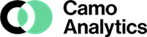제품소개Unscrambler Software

가람교역은 전문화된 인력, 장비, 다양한 분야의 축적된 경험을 바탕으로
사용자가 원하는 모든 자료와 정보를 제공해드립니다

### Unscrambler## APPLICATIONSUnscrambler X ver11.0
= Features =
● Powerful multivariate analysis methods and design of           experiment.
● Easy data importing options with intuitive workflows              and interface.
● Outstanding graphics, plots and interactive data                     visualization tools.

= Explorative Methods =
● PCA ( Principal Component Analysis )
● MCR ( Multivariate Curve Resolution )

= Regression Methods =
● MLR (Multiple Linear Regression )
● PCR ( Principal Component Regression )
● PLSR ( Partial Least Squares Regression )
● SVR ( Support Vector Machines Regression )
● L-PLS Regression, incorporating three data tables.

= Classification Methods =
● Projection using PCA, PCR or PLSR models.
● SIMCA ( Soft Independent Modelling of Class Analogy )
● LDA ( Linear Discriminant Analysis ) with Linear,                        Quadratic, Mahalonobis options.
● PCA-LDA, for classification of correlated data by LDA.
● SVC ( Support Vector Machines Classification ).